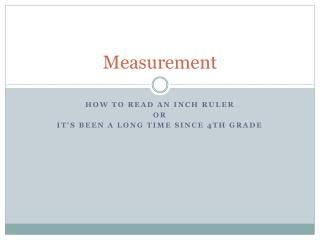DownloadDownload PresentationMeasurement

# Measurement

Télécharger la présentation## Measurement

- - - - - - - - - - - - - - - - - - - - - - - - - - - E N D - - - - - - - - - - - - - - - - - - - - - - - - - - -
##### Presentation Transcript

1. Measurement HOW TO READ AN INCH RULER OR It’s been a long time since 4th grade

2. What measurement is this?

4. What you need to know This is 1/16 inch ruler.We know this because it has 16 white spaces in one inch.

5. What you need to know This is 1/16 inch ruler.We know this because it has 16 white spaces in one inch. This slide shows one inch.

6. Answer: 1/2 This is 1/16 inch ruler.We know this because it has 16 white spaces in one inch. This slide shows one inch. The size of the line it is pointing to gives you a hint as to what the measurement is. The longer the line the bigger the measurement. 1/2

7. Answer: 1/2 This is 1/16” ruler—we know this because it has 16 white spaces in it it shows one inch The size of the line it is pointing to gives you a hint as to what the measurement is The longest line on a ruler shows the inch mark, next longest shows the ½ inch mark, next longest shows the ¼. etc Fractions must always be reduced i.e. 1/8 not 2/16 1/8

8. What measurement is this

10. What measurement is this

12. What measurement is this

14. What measurement is this

16. What measurement is this

18. What measurement is this

20. What measurement is this

22. What measurement is this

24. Measurement HOW TO READ A pica gauge OR WOW! look at me acting like a graphic designer

25. Measuring with points and picas

26. Points converted to picas • Measurements: • 12 points =1 pica • 6 picas =1 inch • 72 points =1 inch • ½ pica= 6 points • A standard US letter size page is 8.5 by 11 inchesor 51 by 66 picas. p12 1p

27. Picas converted to inches • Measurements: • 12 points =1 pica • 6 picas =1 inch • 72 points =1 inch • ½ pica= 6 points • A standard US letter size page is 8.5 by 11 inchesor 51 by 66 picas. 6p 1 inch

28. Points converted to inches • Measurements: • 12 points =1 pica • 6 picas =1 inch • 72 points =1 inch • ½ pica= 6 points • A standard US letter size page is 8.5 by 11 inchesor 51 by 66 picas. 12 24 36 48 60 72 points 72 points or I inch

29. points | picas • Measurements: • 12 points =1 pica • 6 picas =1 inch • 72 points =1 inch • ½ pica= 6 points • A standard US letter size page is 8.5 by 11 inchesor 51 by 66 picas. 6 12 18 24 points ½ pica or 6 points

30. What measurement is this

31. Answer 8p9 | 8 picas 9 points

32. What measurement is this

33. Answer 1p | 1 picas 0 points

34. What measurement is this

35. Answer 1p6 | 1 picas 6 points

36. What measurement is this

37. Answer 2p3 | 2 picas 3 points